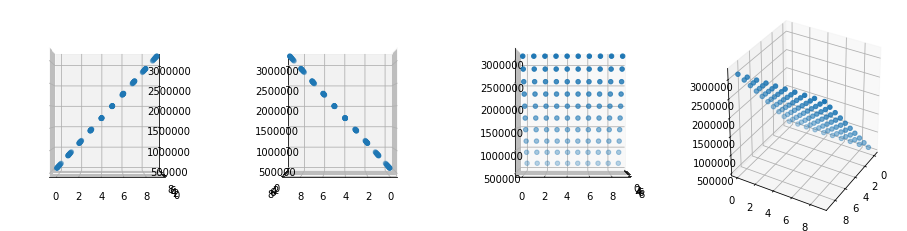# Advent of Code 2019 Day 2¶

I ended up awake near the time problem was about to release, and decided to give it a shot: I was ranked 910/1502, mostly because I skimmed the questions too aggressively. The published notebook is a more cleaned up version of the code, particularly because we can expect more opcode based problems.

In :
input = []
with open("AoC/input2.txt") as input_file:


The first problem is direct enough: opcode based turing machine where I can stop forward and evaluate the program.

In :
def compute(program):
l = len(program)
i = 0
while i < l:
op = program[i]
if op == 99:
return program

a, b, out = program[i + 1: i + 4]

if op == 1:
program[out] = program[a] + program[b]
elif op == 2:
program[out] = program[a] * program[b]

i += 4

In :
compute([int(x) for x in "1,9,10,3,2,3,11,0,99,30,40,50".split(",")])

Out:
[3500, 9, 10, 70, 2, 3, 11, 0, 99, 30, 40, 50]
In :
compute([1, 0, 0, 0, 99])

Out:
[2, 0, 0, 0, 99]
In :
compute([2, 3, 0, 3, 99])

Out:
[2, 3, 0, 6, 99]
In :
compute([2, 4, 4, 5, 99, 0])

Out:
[2, 4, 4, 5, 99, 9801]
In :
compute([1, 1, 1, 4, 99, 5, 6, 0, 99])

Out:
[30, 1, 1, 4, 2, 5, 6, 0, 99]

I wasted a lot of time by forgetting that the input list was being mutated by compute, which cost me several precious minutes to debug.

In :
new_input = list(input)
new_input = 12
new_input = 2
compute(new_input)

Out:
3931283

## Part 2¶

For the second problem, I missed the specifications that the inputs were in the range [0, 99]: I started by making a 3D plot of the x,y,z values (from 0 - 100).

In :
def compute_inputs(x, y):
new_input = input[:]
new_input = x
new_input = y
return compute(new_input)

In :
import matplotlib.pyplot as plt
from mpl_toolkits.mplot3d import Axes3D
%matplotlib inline

fig = plt.figure(figsize=(16, 4))
xs, ys, zs = [], [], []
for x in range(10):
for y in range(10):
z = compute_inputs(x, y)
xs.append(x)
ys.append(y)
zs.append(z)

ax.scatter(xs, ys, zs)
ax.view_init(30, 30)

ax2.scatter(xs, ys, zs)
ax2.view_init(0, -90)

ax3.scatter(xs, ys, zs)
ax3.view_init(0, 90)

ax4.scatter(xs, ys, zs)
ax4.view_init(0, 0)

plt.show()This made me realize it was a linear combination of the form z = ax + by + c from the shape. Checking the values at (0, 0), (1, 0), (0, 1) gave me a clear equation.

In :
i = 0
for x in range(2):
for y in range(2):
i += 1
print(f"x: {x}, y: {y}, z: {compute_inputs(x, y)} -- [{i}]")

x: 0, y: 0, z: 613521 -- 
x: 0, y: 1, z: 613522 -- 
x: 1, y: 0, z: 890001 -- 
x: 1, y: 1, z: 890002 -- 


From 1, c = 613521

From 2, b = 613522 - 613521 = 1

From 3, a = 890001 - 613521 = 276480

After that, it was mainly about finding an integer solution to 19690720 - 613521 = 276480 * x + y, which can be calculated by integer division and modulus (of course there are an infinite number of solutions if you allow for negative numbers, but I was just testing for the direct one).

In :
x, y = (19690720 - 613521) // 276480, (19690720 - 613521) % 276480
x, y

Out:
(69, 79)

Sanity checking backwards,

In :
compute_inputs(x, y)

Out:
19690720

Of course, if I had read the problem correctly, this would have been a little more trivial:

In :
next((x, y) for x in range(100) for y in range(100) if compute_inputs(x, y) == 19690720)

Out:
(69, 79)

TODO: At some point, try to do this symbolically, or by representing values as a tuple.